Problem solving 101

Never be stumped by a maths problem again, with this crash course from the ever-competent Stephen Muirhead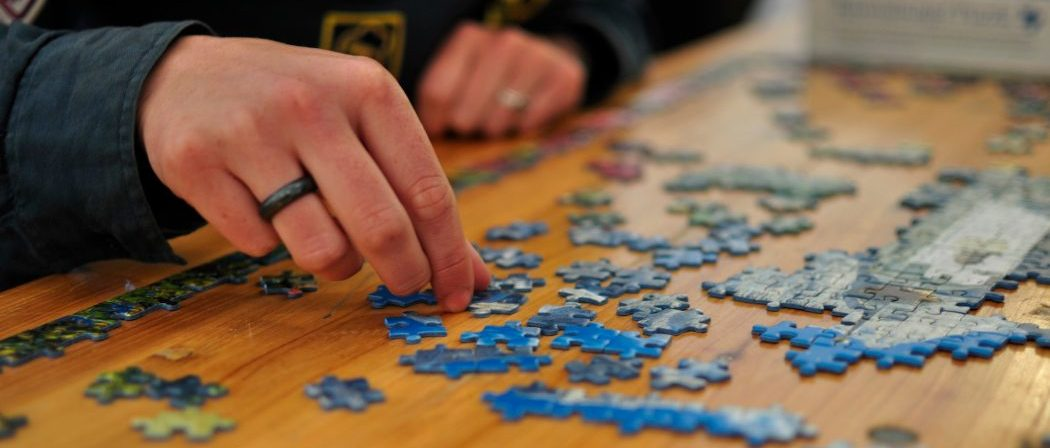From the outside looking in, maths problem solving can seem like a kind of magic. Here is a typical image: a lone genius, peering at a vexing problem, rubs their chin, paces up and down; then a bolt of inspiration hits and the solution falls neatly into place.

And while it’s true that inspiration can strike the lucky few, for the rest of us this is no more than an illusion (and often a carefully cultivated illusion at that). In reality, problem solving is usually much more prosaic, nothing more than a careful application of well-known, and often quite elementary, techniques.

So what are these elementary techniques? In this article, I’ll look at some of the simplest and easiest to understand. Happily, they are also some of the most powerful and widely applicable. These techniques will be explained by way of example problems; I strongly encourage you to attempt the problems yourself before reading the solutions.

Get some intuition

Faced with an unfamiliar problem, it can often be difficult to know where to begin. An important first step is to develop some intuition. This will often allow you to guess the correct answer—an important step in itself—or, if you’re lucky, point you in the direction of a full solution. A good way to develop intuition is to play around with the parameters of the problem, looking for particular easy cases in which the solution is clear. Let’s look at an example.

Puzzle 1

Two people take turns placing identical plates on a square table in such a way that they do not touch; the first who cannot place another plate loses. Which player has a winning strategy?At first glance this problem appears underspecified. How large is the table? What shape and size are the plates? This seems an additional difficulty to surmount. In another light, it also suggests an opportunity to gain intuition, by varying these parameters. For example, suppose the table were very, very small. Then clearly the first player has a winning strategy, because there would only be space for a single plate. Although far from a proof, this gives a strong hint that the first player always has a winning strategy, since otherwise there must be a critical threshold of table size at which the solution to the problem fundamentally changes, and this is rather implausible.

To develop this idea further, you might next consider a table that is only slightly larger than the size of a single plate, and determine a winning strategy in that case. We will see the solution to this problem later. For now, let’s see a second example.

Puzzle 2

Would it be quicker to run along a moving walkway (a travelator) to the end and back again, first with the flow and then against the flow, or to run the same path along the ground?Once again the problem appears underspecified, and once again this suggests a strategy for guessing the answer, by playing around with the parameters. Suppose that the travelator were moving very fast, faster than you could run. Then you could never run successfully against the flow, and so it is certainly quicker to run on the ground. On the other hand, if the travelator had zero speed, then the two routes would take the same amount of time. This strongly hints at the correct answer: that taking the travelator is always slower, unless it is not moving.Find and exploit the structure of the problem

Maths is essentially the study of patterns, and a problem will generally be easier if you can identify and exploit some structure. A common example is symmetry—geometric, algebraic, etc—and exploiting symmetry in an appropriate way often leads to very simple and elegant proofs. Symmetry comes in many varieties, but in general you should look for transformations of the problem (rotation in space, permutation of variables, etc) that leave the important features unchanged.

Problem 3If the outer square on the left has area $1$, what is the area of the inner square?

Given this, calculate the proportion of the figure on the right that is coloured blue.There is strong symmetry in both of the above pictures. Aside from the obvious reflective and rotational symmetries, there are also subtler versions more relevant to the quantities we wish to calculate. In the first image, the area of the inner square does not change if we rotate it, leaving the outer square fixed. Why is this useful? Well, consider rotating the inner square 45 degrees. Then by decomposing the outer square into quarters, shown here on the left, it is clear that the inner square has half the area of the outer square.

In the problem’s second picture, notice that the same image is repeated at a smaller scale inside the inner square: this can be thought of as nested symmetry. This implies that the proportion of the whole square that is coloured blue is the same as the proportion of the inner square that is coloured blue. Combining this with the first observation, we come to the equation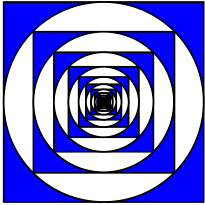Total blue area = Blue area in outer ring + $1/2$ $\times$ Total blue area

In other words,

Total blue area = 2 $\times$ Blue area in outer ring.

Since the blue area in the outer ring is $1-\mathrm{\pi}/4$ (subtract the area of the circle from the area of the square), and the area of the whole square is 1, the proportion of blue in the figure is $2 – \mathrm{\pi}/2$.

Problem 4

Calculate the values of

$1 + \frac{1}{1 + \frac{1}{1 + \frac{1}{1 + \cdots}}} \quad \text{and} \quad 2 + \sqrt{2 + \sqrt{2 + \sqrt{2 + \cdots}}}$.

These expressions look completely different to the squares and circles we saw in the previous problem, but mathematically the problems have a similar structure, just in an algebraic rather than geometric setting. In particular, they have nested symmetry that we can exploit in the same way as before. Let the values we wish to calculate be $X$ and $Y$. Then, exploiting the nested symmetry, $X$ and $Y$ must satisfy the equations

$X = 1 + \frac{1}{\!X}$    and    $Y = 2 + \sqrt{Y}$.

(Some care is needed to justify working with the limits, but we will ignore this point.) These equations are now easy to solve, giving the values

$X = \frac{1 + \sqrt{5}}{2}$    and    $Y = 4$.

Problem 5

How soon after midnight do a clock’s hour and minute hands first meet?

This problem can be readily solved using simultaneous equations, but we can also exploit the symmetry of the clock to give a simpler solution. Imagine watching the clock for a full 12 hours from midnight until midday, and notice that the hands start together and end together. Between these times, the symmetry of the clock implies that the times at which the clock hands meet are evenly spaced. To see this, consider the moment when the hands first meet and imagine rotating the clock face until the hands are upright. Then, the movements of the clock hands from this moment on are identical to their movements from midnight, and so the situation is symmetrical.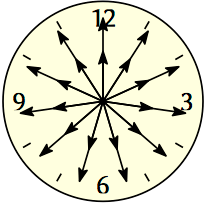How many times do the hands meet between midnight and midday? Well, the hour hand goes once round the clock in this period, and the minute hand goes round 12 times. Hence they meet 11 further times between midnight and midday inclusive, and the first time they meet is therefore

Midnight + $\frac{1}{11} \times 12$ hours $\approx$ 1.05 am.Finally, let’s return to the problem involving placing plates on a square table (Problem 1), and use symmetry to give the first player a winning strategy. Such a strategy needs to ensure that, no matter the current state of the table, if the second player can place a plate, the first player can also do so. This suggests that a strategy of “copying” the moves of the second player might work. Indeed, if the first player places the first plate in the dead centre of the table, they can play any further moves by rotating the second player’s most recent move by 180 degrees. It’s easy to check that this guarantees a win for the first player.

Look for quantities that do not change

A question that often perplexes newcomers to mathematics is how to go about proving that something does not exist, or that a procedure is impossible. Of course, proving that something exists (for example, an integer $x$ such that $x^2 = 144$) is simple: just construct it explicitly. But to prove the opposite would seem to require checking an infinite number of possibilities. The technique of finding quantities that do not change—invariants—or more generally quantities that change in a controlled manner—monovariants—gives rise to elegant and simple solutions to problems of this sort. Let’s see a typical example.

Problem 6

Prove that a $5 \times 13$ rectangle cannot be cut up into pieces and rearranged into an $8 \times 8$ square.You could attempt all the different ways of cutting up the rectangle, and show that none of them can be arranged into the square. It wouldn’t take long to realise that the number of ways is infinite. Instead, we construct a quantity that doesn’t change, no matter how the rectangle is cut up. If this quantity is different for the rectangle and the square, we will have shown that no cutting procedure can change one into the other. The total area of a shape gives a natural invariant: when a piece is cut in two, no matter how, the total area stays the same. Since the rectangle’s area is 65 and the square’s is 64, we have proved that the task cannot be done.

Apart from proving that things are impossible, invariants can also be used to show that a given procedure always produces the same result,
no matter how it is undertaken. Let’s see an example.

Problem 7

Suppose that the numbers 1 to 20 are written on a blackboard. At each turn, you may erase two numbers $a$ and $b$ and write the sum $a+b$ in their place. After 19 such turns, there will be one number left on the board. What will this number be?

If you try this a few times, you’ll soon see that the final number is 210 no matter which way you do the erasing. To prove this, notice that there is a simple quantity that does not change no matter how you erase: the total sum of all the numbers currently written on the board. Hence, the total sum at the end of the process—which is just the final number—must be equal to the total sum of the numbers at the start of the process, which is $1 + 2 + \cdots + 20 = 210$.

A monovariant, which changes in a predictable manner, might be a quantity that always increases, or one that always alternates between 0 and 1. Consider the following example.

Problem 8

Three coins lie well spaced out on a table. Each turn, you flick one of the coins so that it passes between the other two without touching them. If you do this 99 times, is it possible for you to return each of the coins to their original positions?

Suppose we try to return the coins to their original positions and fail each time. Could we prove it is impossible? It’s not obvious how to use an invariant here: we are asking precisely that the coins end up in an identical position, and so it seems that no invariant could prove this impossible. But remark that we want to return the coins after an odd number of flicks. This suggests looking for a quantity that always alternates between two values, say from 0 to 1 and then back to 0, etc. If we do this, we will have proved the impossibility of returning the coins after 99 flicks.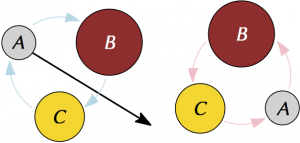Such a quantity is the cyclic ordering of the coins: in other words, if the coins are labelled $A$, $B$ and $C$, it is either the ordering $ABC$ or $ACB$, depending on which way the labels go when taken in a clockwise order. When we make a flick, this cyclic order always switches. Hence after 99 flicks the coins will lie in a different cyclic order, and so cannot be in their original positions.

In all these examples the invariant/monovariant was very intuitive, but often this will not be the case. A big part of the art is finding an appropriate invariant. Consider the following problem, which is one of my favourites.

Problem 9Three amoebae are placed at positions $(0, 0)$, $(1, 0)$ and $(0, 1)$ on a two-dimensional lattice. Each turn, one of the amoebae reproduces by splitting in two, with one offspring moving to the site directly right and the other offspring moving to the site directly above, but only if both of these sites are currently vacant (if they are not, the amoeba stays put).

Is it possible that eventually the three initial sites will all be free of amoebae?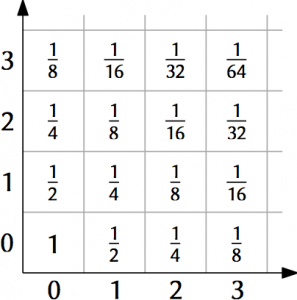A quick play around may convince you that this is impossible—the grid gets clogged up pretty quickly—but it is very hard to be sure. To prove this conclusively, we construct a quantity that does not change, and show that vacating the three initial sites would imply a change in this quantity. The idea is to assign a score to each square that contains an amoeba. Since the amoebae split in two, and the offspring occupy the squares to the right and directly above, it is natural to score the board with a geometric series, as shown on the left. This ensures that the total score of all the amoebae never changes.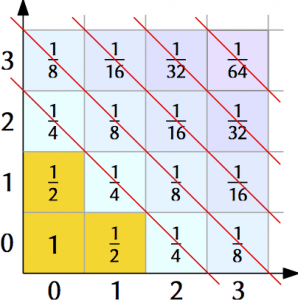Now consider that the initial score is $1 + 1/2 + 1/2 = 2$. What would be the final score, if we were able to vacate the three initial sites? Well, clearly the final score must be strictly less than the total sum of scores on the board outside the three initial sites. Summing up over the whole board as on the right, this number is

$x = \frac{3}{4} + \frac{4}{8} + \frac{5}{16} + \frac{6}{32} + \cdots$.

There are several ways to compute the value of $x$, for instance by decomposing it as

\begin{align*} 2x & = \frac{3}{2} + \frac{4}{4} + \frac{5}{8} + \frac{6}{16} + \cdots \\ &= \frac{3}{2} + \left(\frac{3}{4} + \frac{4}{8} + \frac{5}{16} + \cdots\right) + \left(\frac{1}{4} + \frac{1}{8} + \frac{1}{16} + \cdots\right) \\ & = \frac{3}{2} + x + \frac{1}{2}\left(\frac{1}{2} + \frac{1}{4} + \frac{1}{8} + \cdots\right), \end{align*}

and using the formula

$1 = \frac{1}{2} + \frac{1}{4} + \frac{1}{8} + \cdots,$

to get that the total score, $x$, is exactly 2, precisely the value of the initial score. So we have a contradiction (but only just!).

Consider the extremes

The final technique we will look at involves extremes: that is, mathematical objects that are the biggest, smallest, quickest, etc. There are two essentially distinct ways in which extremes are useful in problem solving. The first is to prove that something cannot exist, arguing by contradiction as follows. Begin by assuming the thing exists, and deduce that there must be a most extreme version of that object (note that this may not always be the case: there is no largest prime, for example). Then, use this to construct a version that is even more extreme, resulting in a contradiction. Let’s see a classic example.

Problem 10

Prove that $\sqrt{2}$ is irrational, ie no integers $p$ and $q$ exist such that $\sqrt{2} = p/q$.

We argue by contradiction. Suppose that $\sqrt{2}$ were rational and hence could be written as a fraction. Then consider the fraction in its reduced form: that is, the fraction $p/q$ such that $p$ and $q$ are integers with no common factor (this is the most extreme version of the fraction, in this context). Now we deduce that

$\sqrt{2} = \frac{p}{q} \implies p^2 = 2 q^{\,2}$,

which implies that $p$ is a multiple of $2$. Substituting $p = 2r$, we get

$(2r)^2 = 2q^{\,2} \implies q^{\,2} = 2 r^{\,2}$,

which implies that $q$ is also a multiple of $2$. Hence the fraction $p/q$ was not in reduced form (ie we can find a fraction that is even more reduced), which establishes the contradiction.

The second way in which extremal reasoning is useful is to prove that something does exist, by constructing it explicitly. In order to come up with a construction, it is usually helpful to start with extremal objects, and base the construction around them.

Problem 11

In a `round robin’ tennis tournament of $N \geq 3$ players, each player plays each other exactly once. If no one won all their games, prove that we can find three players such that Player A beat Player B, Player B beat Player C, and Player C beat Player A.

The tricky thing about this problem is that there are many ways the tournament could have played out, and it is difficult to know where to look for players with the desired property. One idea is to base the construction around the player that won the most games, the extremal player in this context—call this Player A (if there are several such players, just pick one of them arbitrarily).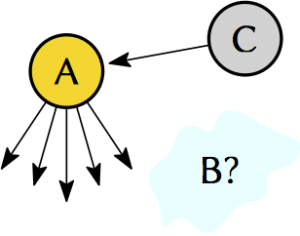What do we know about Player A? Well, certainly they beat some of the players (in fact, at least half). Also, because no player won all their games, at least one player beat Player A: pick one of these and call them Player C. Now consider all the players that Player A beat. All that is left is to deduce that at least one of them also beat Player C, because then this player can be Player B. But this must be true, because if it were not, Player C would have beaten more players than Player A (all the players that Player A beat, plus Player A), contradicting the assumed extremality of Player A.

Problem solving 102

With some practice, the above basic techniques will get you a fair way in being able to tackle a variety of maths problems. But they are by no means the end of the story. Beyond these, there are many other general techniques that are widely applicable and extremely useful; a good reference is Paul Zeitz’s The Art and Craft of Problem Solving, from which several of the problems in this article have been taken. Of course, each subfield of mathematics also has its own techniques that are more or less specific to it. One of the great pleasures of maths is that the well of techniques is very deep, and hopefully is a long way from running dry.

[Banner image: Wikimedia user Scouten, CC-BY-SA 3.0. Other images: Chalkdust.]Stephen is a lecturer in mathematics at Queen Mary University of London. Like any mathematician, he is always delighted to find unexpected connections between mathematical objects.
stephenmuirhead.wordpress.com    + More articles by Stephen